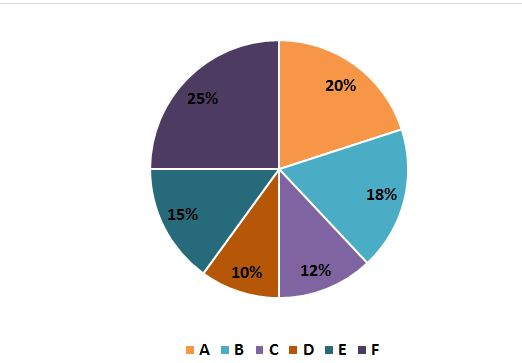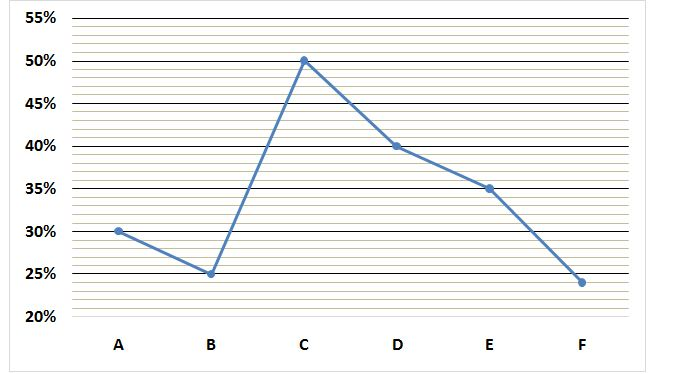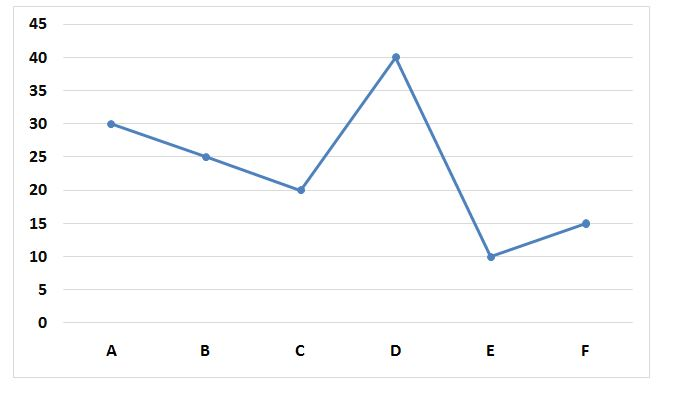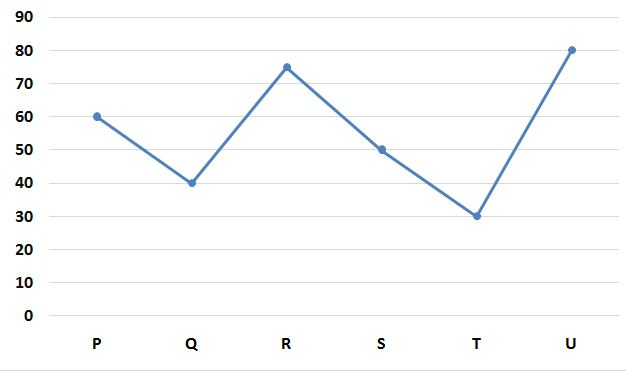# SBI PO Quantitative Aptitude Questions 2019 (Day-27) High Level New Pattern

SBI PO 2019 Notification is about to come and it is the most awaited exam among the aspirants. We all know that new pattern questions are introducing every year in the SBI PO exam. Further, the questions are getting tougher and beyond the level of the candidate’s expectations.

Our IBPS Guide is providing High-Level New Pattern Quantitative Aptitude Questions for SBI PO 2019 so the aspirants can practice it on a daily basis. These questions are framed by our skilled experts after understanding your needs thoroughly. Aspirants can practice these high-level questions daily to familiarize with the exact exam pattern. We wish that your rigorous preparation leads you to a successful target of becoming SBI PO.

[WpProQuiz 5368]

### Click Here for SBI PO Pre 2019 High-Quality Mocks Exactly on SBI Standard

Directions (1 – 5): Study the following information carefully and answer the questions given below:

The following pie chart represents percentage wise distribution of quantity of mixture of wine and water in six containers.

Total quantity of mixture of wine and water in six containers = 1200 litres.The following line graph represents percentage of water in six containers.1) 25% of the mixture of wine and water in container D is used in a party and 16 litres of pure wine and 14 litres of water added to the remaining mixture of that container. If 24 litres of the mixture is again used and 4 litres of pure wine added to the container, the quantity of wine in the initial mixture of container D will be what percent of quantity of wine in the final mixture of that container?

a) 95%

b) 100%

c) 120%

d) 80%

e) None of these

2) If 40% mixture of container A, 25% mixture of container C and 30% mixture of container E are mixed together in container G which contains 40 litres of pure wine and 25 litres of water, find the respective ratio of wine and water in the final mixture of container G?

a) 903 : 503

b) 1603 : 907

c) 1287 : 503

d) 1102 : 607

e) None of these

3) Mixtures of container B and container F are mixed together in container H which was empty. 4 litres of water and 10 litres of pure wine added into it. 53 litres of the mixture is used. Find the difference between the quantity of wine and quantity of water in the remaining mixture of container H?

a) 157 litres

b) 243 litres

c) 197 litres

d) 311 litres

e) None of these

4) Find the relation between following two quantities:

Quantity I: 40 % of the mixture in container A is used and remaining mixture of container A is transferred to container F. Find the total quantity of wine in container F.

Quantity II: 20 % of the mixture in container B is used and remaining mixture of container B is transferred to container E. Find the total quantity of wine in container E.

a) Quantity I > Quantity II

b) Quantity I < Quantity II

c) Quantity I ≥ Quantity II

d) Quantity I ≤ Quantity II

e) Quantity I = Quantity II or no relation can be established

5) Find the difference between quantity of wine and quantity of water in containers C and G together?

Statement I: Quantity of wine in container G is 25% more than the quantity of wine in container D.

Statement II: Total quantity of mixture in container G is 10% more than the total quantity of mixture in container F.

a) Statement I alone is sufficient to answer the question, but the statement II alone is not sufficient.

b) Statement II alone is sufficient to answer the question, but the statement I alone is not sufficient.

c) Either statement I alone or statement II alone is sufficient to answer the question.

d) Both statements I and II together are needed to answer the question.

e) Both statements I and II together are not sufficient to answer the question.

Directions (6 – 10): Study the following information carefully and answer the questions given below:

The first line graph represents time taken (in minutes) by six pipes to fill the cistern.The second line graph represents time taken (in minutes) by six pipes to empty the cistern.6) Pipe A and pipe C are opened simultaneously and closed after 5 minutes. Then, pipe B and pipe D are opened simultaneously. Pipe B is closed before 5 minutes of filling the cistern. Find the time for which pipe B was opened?

a) 215/37 days

b) 185/43 days

c) 275/39 days

d) 290/41 days

e) None of these

7) Pipe E, pipe Q and pipe F are opened simultaneously for 4 minutes and closed. Then, pipe P and pipe U are opened for 10 minutes and closed. Find the time taken by pipe A and pipe E to fill the remaining part of the cistern?

a) 85/8 days

b) 81/15 days

c) 79/13 days

d) 75/11 days

e) None of these

8) Find the respective ratio of the time taken by pipe B, pipe D and pipe F to fill the cistern, time taken by pipe P, pipe R and pipe T to empty the cistern and time taken by pipe A, pipe C and pipe T to fill the cistern?

a) 511 : 1235 : 1721

b) 570 : 1185 : 1501

c) 413 : 1086 : 1121

d) 619 : 1175 : 1321

e) None of these

9) If Pipe G and H are inlet pipes, then

Quantity I: Pipe D, pipe U and pipe G together can fill the cistern in 16 minutes. Find the time taken by pipe P, pipe G and pipe E to fill the cistern.

Quantity II: Efficiency of pipe H is 20% more than the efficiency of pipe A. Find the time taken by pipe E, pipe H and pipe Q to fill the cistern.

a) Quantity I > Quantity II

b) Quantity I < Quantity II

c) Quantity I ≥ Quantity II

d) Quantity I ≤ Quantity II

e) Quantity I = Quantity II or no relation can be established.

10) Find the time taken by pipe F, pipe R and pipe K to fill one fourth part of the cistern, if pipe K and M are inlet pipes?

Statement I: Pipe K, pipe M and pipe T together can fill the cistern in 60/7 minutes.

Statement II: Pipe M, pipe A and pipe F together can fill the cistern in 20/3 minutes.

a) Statement I alone is sufficient to answer the question, but the statement II alone is not sufficient.

b) Statement II alone is sufficient to answer the question, but the statement I alone is not sufficient.

c) Either statement I alone or statement II alone is sufficient to answer the question.

d) Both statements I and II together are needed to answer the question.

e) Both statements I and II together are not sufficient to answer the question.

Directions (1-5):

Total quantity of mixture in container D = (10/100) x 1200 = 120 litres

Initial quantity of water in container D = (40/100) x 120 = 48 litres

Initial quantity of wine in container D = 120 – 48 = 72 litres

Wine : Water = 72 : 48 = 3 : 2

Quantity of wine remained in the container after using 25% of the mixture

= 72 – (3/5) x (25/100) x 120

= 72 – 18 = 54 litres

Quantity of water remained in the container after using 25% of the mixture

= 48 – (2/5) x (25/100) x 120

= 48 – 12 = 36 litres

Now,

Wine : Water = (54 + 16) : (36 + 14)

= 70 : 50 = 7 : 5

Quantity of wine in the final mixture = 70 – 7/12 x 24 + 4

= 70 – 14 + 4

= 60 litres

Required percentage = (72/60) x 100 = 120%

Total quantity of mixture in container A = (20/100) x 1200 = 240 litres

Quantity of water in container A = (30/100) x 240 = 72 litres

Quantity of wine in container A = 240 – 72 = 168 litres

Wine : Water = 168 : 72 = 7 : 3

Total quantity of mixture in container C = (12/100) x 1200 = 144 litres

Quantity of water in container C = (50/100) x 144 = 72 litres

Quantity of wine in container C = 144 – 72 = 72 litres

Wine : Water = 72 : 72 = 1 : 1

Total quantity of mixture in container E = (15/100) x 1200 = 180 litres

Quantity of water in container E = (35/100) x 180 = 63 litres

Quantity of wine in container E = 180 – 63 = 117 litres

Wine : Water = 117 : 63 = 13 : 7

Respective ratio of wine and water in the final mixture of container G

= (40 + 7/10 x 40/100 x 240 + ½ x 25/100 x 144 + 13/20 x 30/100 x 180) : (25 +  3/10 x 40/100 x 240 + ½ x 25/100 x 144 + 7/20 x 30/100 x 180)

= (40 + 67.2 + 18 + 35.1) + (25 + 28.8 + 18 + 18.9)

= 160.3 : 90.7

= 1603 : 907

Total quantity of mixture in container B = (18/100) x 1200 = 216 litres

Quantity of water in container B = (25/100) x 216 = 54 litres

Quantity of wine in container B = 216 – 54 = 162 litres

Total quantity of mixture in container F = (25/100) x 1200 = 300 litres

Quantity of water in container F = (24/100) x 300 = 72 litres

Quantity of wine in container F = 300 – 72 = 228 litres

In container H:

Wine = 162 + 228 + 10 = 400 litres

Water = 54 + 72 + 4 = 130 litres

Wine : Water = 400 : 130 = 40 : 13

Quantity of wine in the remaining mixture of container H

= 400 – 40/53 x 53 = 360 litres

Quantity of water in the remaining mixture of container H

= 130 – 13/53 x 53 = 117 litres

Required difference = 360 – 117 = 243 litres

Quantity I:

Total quantity of mixture in container A = (20/100) x 1200 = 240 litres

Quantity of water in container A = (30/100) x 240 = 72 litres

Quantity of wine in container A = 240 – 72 = 168 litres

Wine : Water = 168 : 72 = 7 : 3

Total quantity of mixture in container F = (25/100) x 1200 = 300 litres

Quantity of water in container F = (24/100) x 300 = 72 litres

Quantity of wine in container F = 300 – 72 = 228 litres

Total quantity of wine in container F

= 228 + 240 x (7/10) x [(100 – 40)/100]

= 228 + 240 x 7/10 x 60/100

= 228 + 100.8

= 328.8 litres

Quantity II:

Total quantity of mixture in container B = (18/100) x 1200 = 216 litres

Quantity of water in container B = (25/100) x 216 = 54 litres

Quantity of wine in container B = 216 – 54 = 162 litres

Wine : Water = 162 : 54 = 3 : 1

Total quantity of mixture in container E = (15/100) x 1200 = 180 litres

Quantity of water in container E = (35/100) x 180 = 63 litres

Quantity of wine in container E = 180 – 63 = 117 litres

Total quantity of wine in container E = 117 + ¾ x 216 x [(100 – 20)/100]

= 117 + 216 x ¾ x 80/100

= 117 + 129.6

= 246.6 litres

Hence, Quantity I > Quantity II

Total quantity of mixture in container C = (12/100) x 1200 = 144 litres

Quantity of water in container C = (50/100) x 144 = 72 litres

Quantity of wine in container C = 144 – 72 = 72 litres

From I:

Total quantity of mixture in container D = (10/100) x 1200 = 120 litres

Quantity of water in container D = (40/100) x 120 = 48 litres

Quantity of wine in container D = 120 – 48 = 72 litres

Quantity of wine in container G = 72 x (125/100) = 90 litres

From II:

Total quantity of mixture in container F = (25/100) x 1200 = 300 litres

Total quantity of mixture in container G = 300 x (110/100) = 330 litres

From I and II:

Quantity of water in container G = 330 – 90 = 240 litres

Required difference = (72 + 240) – (72 + 90)

= 312 – 162

= 150 litres

Hence, both the statements I and II are needed to answer the question.

Directions (6-10):

Part of the cistern filled by pipe A and pipe C = 5/30 + 5/20

= 1/6 + 1/4

= (2 + 3)/12

= 5/12

Remaining part of the tank = 1 – 5/12

= (12 – 5)/12

= 7/12

Let the time taken by B be ‘n’ minutes,

n/25 + (n + 5)/40 = 7/12

= > (8n + 5n + 25)/200 = 7/12

= > 13n + 25 = 200 x 7/12

= > 13n = (350/3) – 25

= > 13n = (350 – 75)/3

= > 13n = 275/3

= > n = (275/3) x (1/13)

= > n = 275/39 days

Part of the tank filled by pipe E, pipe Q and pipe F in 4 minutes

= 4/10 – 4/40 + 4/15

= (48 – 12 + 32)/120

= 68/120

= 17/30

Part of the tank emptied by pipe P and pipe U in 10 minutes = 10/60 + 10/80

= (40 + 30)/240

= 70/240

= 7/24

Remaining part of the tank = (1 – 17/30) + (17/30 – 7/24)

= (30 – 17)/30 + (408 – 210)/(30*24)

= 13/30 + 198/720 = (312 + 198)/720

= 510/720

= 17/24

Let, required time = n minutes

n/30 + n/10 = 17/24

= > (n + 3n)/30 = 17/24

= > 4n/30 = 17/24

= > n = (30/4) x (17/24)

= > n = 85/8 days

Let, time taken by pipe B, pipe D and pipe F to fill the cistern = n minutes

Time taken by pipe P, pipe R and pipe T to empty the cistern = k minutes

And time taken by pipe A, pipe C and pipe T to fill the cistern = t minutes

n x (1/25 + 1/40 + 1/15) = 1

=> n x (24 + 15 + 40)/600 = 1

=> n = 600/79 minutes

k x (1/60 + 1/75 + 1/30) = 1

=> k x (5 + 4 + 10)/300 = 1

=> k = 300/19 minutes

t x (1/30 + 1/20 – 1/30) = 1

=> t/20 = 1

=> t = 20 minutes

Required ratio = 600/79 : 300/19 : 20

= 570 : 1185 : 1501

Quantity I:

1/D – 1/U + 1/G = 1/16

=> 1/40 – 1/80 + 1/G = 1/16

=> 1/G = 1/16 – 1/40 + 1/80

=> 1/G = (5 – 2 + 1)/80

=> 1/G = 4/80

=> 1/G = 1/20

Let, required time = n minutes

n x (-1/60 + 1/20 + 1/10) = 1

= > n x (-1 + 3 + 6)/60 = 1

= > n = 60/8

= > n = 15/2 minutes

Quantity II:

1/H = 1/30 x (120/100)

=> 1/H = 1/25

Let, required time = t minutes

t x (1/10 + 1/25 – 1/40) = 1

=> t x (20 + 8 – 5)/200 = 1

=> t = 200/23 minutes

Hence, Quantity I < Quantity II

From I:

1/K + 1/M + 1/T = 7/60

=> 1/K + 1/M – 1/30 = 7/60

=> 1/K + 1/M = 7/60 + 1/30

=> 1/K + 1/M = (7 + 2)/60

=> 1/K + 1/M = 9/60

=> 1/K + 1/M = 3/20

From II:

1/M + 1/A + 1/F = 3/20

=> 1/M + 1/30 + 1/15 = 3/20

=> 1/M + (1 + 2)/30 = 3/20

=> 1/M + 3/30 = 3/20

=> 1/M + 1/10 = 3/20

=> 1/M = 3/20 – 1/10

=> 1/M = (3 – 2)/20

=> 1/M = 1/20

From I and II:

1/K + 1/20 = 3/20

=> 1/K = 3/20 – 1/20

=> 1/k = (3 – 1)/20

=> 1/K = 2/20

=> 1/k = 1/10

Let, required time = n minutes

n x (1/15 – 1/75 + 1/10) = ¼

=> n x (10 – 2 + 15)/150 = ¼

=> n = ¼ x 150/23

=> n = 75/46 minutes

Hence, both statements I and II together are needed to answer the question.